Like   Tweet
 /* styles */ July 1, 2020 Dear Readers, Welcome to the eleventh installment of DLPP’s “Expanding Your Horizons While Sheltering at Home” series. A number of writers in 2009 took us back in time and far from home as a means of reflecting on war, social injustice, and a path to peace. We were transported to the Italian mountains during WWII, to North Dakota reservation communities torn by racism, to the Cuban sugar plantations of the late 1950’s. In the days before the Civil war, we watched a runaway slave and his pursuer wade the swamps of Maryland’s eastern shore, we learned that pacifism might have had a fighting chance prior to WWII, and we learned how tribes became nations from one of Dayton’s own, Strobe Talbot. Other writers brought the current world right into our homes: stories of African children torn by poverty and violence are read in juxtaposition with a book on Africa’s corruption stemming from exploitation by the west. We learn of the current issues of climate change, human trafficking, a war-torn Iraq, a man’s reawakening after a decade-long coma to relive the horrors of Tiananmen Square, and strife between Israel and Lebanon. Stories familiar to us still. As I looked back through the titles, I realized that we are separated by more than a decade from the publication of these books and yet they still ring true. For those of you who joined the DLPP family in more recent years, there is gold in these books—books that have already stood the test of time. Stay safe. Be well. Read books.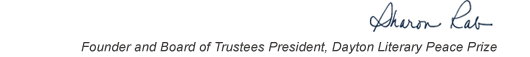table div table+table+table+table div table{width:100%;padding:0}table div table+table+table+table div table img{width:96.23%;padding:0;float:none}table div table+table+table+table div table td{width:100%;padding:0 1.88% 18px}/* styles */This list includes the winners and runners-up for 2009 and we have rich background information on each one on our website.

▪ Under Past Winners. scroll down to the year and click on it.
▪ If you click on the winners and runners-up, you will find the author’s photo and bio, a selection from the book, the judges’ citation, the writer’s reflection on literature and peace, and the option of watching the introduction and acceptance speech. If you click on the finalists, you will find a short description of each book.
 ▪ Under Past Winners. scroll down to the year and click on it.
 ▪ If you click on the winners and runners-up, you will find the author’s photo and bio, a selection from the book, the judges’ citation, the writer’s reflection on literature and peace, and the option of watching the introduction and acceptance speech. If you click on the finalists, you will find a short description of each book.

On the bottom right of the home page, you can click on COREScholar, which is built by the Chair of our Curriculum Committee Carol Loranger, Associate Dean of the College of Liberal Arts at Wright State, and maintained for us by Wright State University. There you can find interviews, TED Talks, articles, other books, films, etc. by and about each of our winning and runner-up authors. It is a great resource for students, book clubs, and readers who would like to explore an author in depth.

 table div table+table+table+table+table+table div table{width:100%;padding:0}table div table+table+table+table+table+table div table img{width:96.23%;padding:0;float:none}table div table+table+table+table+table+table div table td{width:100%;padding:0 1.88% 18px}/* styles *//* styles */ “Read global; buy local.” — Marlon James, DLPP 2009 Fiction Winner for The Book of Night Women
 table div table+table+table+table+table+table+table+table div table{width:100%;padding:0}table div table+table+table+table+table+table+table+table div table img{width:96.23%;padding:0;float:none}table div table+table+table+table+table+table+table+table div table td{width:100%;padding:0 1.88% 18px}/* styles */## 2009 Finalists - Fiction

 table div table+table+table+table+table+table+table+table+table+table div table{width:100%;padding:0}table div table+table+table+table+table+table+table+table+table+table div table img{width:96.23%;padding:0;float:none}table div table+table+table+table+table+table+table+table+table+table div table td{width:100%;padding:0 1.88% 18px}/* styles */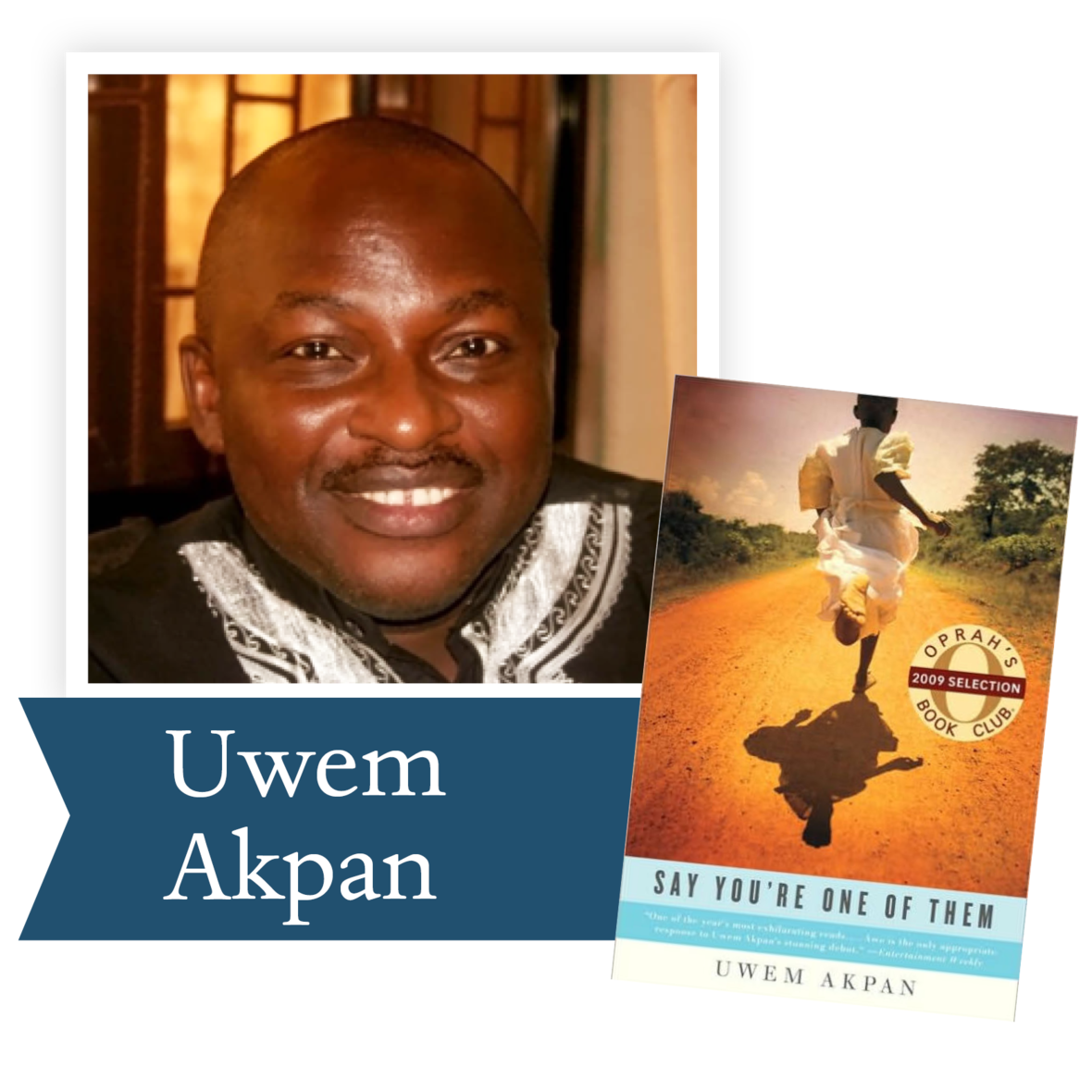Say You’re One of Them by Uwem Akpan (Little, Brown & Company): A Nigerian-born Jesuit priest, Akpan humanizes the perils of poverty and violence facing children in Africa in this stunning collection of five short stories.

 table div table+table+table+table+table+table+table+table+table+table+table+table div table{width:100%;padding:0}table div table+table+table+table+table+table+table+table+table+table+table+table div table img{width:96.23%;padding:0;float:none}table div table+table+table+table+table+table+table+table+table+table+table+table div table td{width:100%;padding:0 1.88% 18px}/* styles */Peace by Richard Bausch (Knopf): Set among American soldiers in Italy during World War Two, Peace is a compelling meditation on the moral dimensions of warfare.

 table div table+table+table+table+table+table+table+table+table+table+table+table+table+table div table{width:100%;padding:0}table div table+table+table+table+table+table+table+table+table+table+table+table+table+table div table img{width:96.23%;padding:0;float:none}table div table+table+table+table+table+table+table+table+table+table+table+table+table+table div table td{width:100%;padding:0 1.88% 18px}/* styles */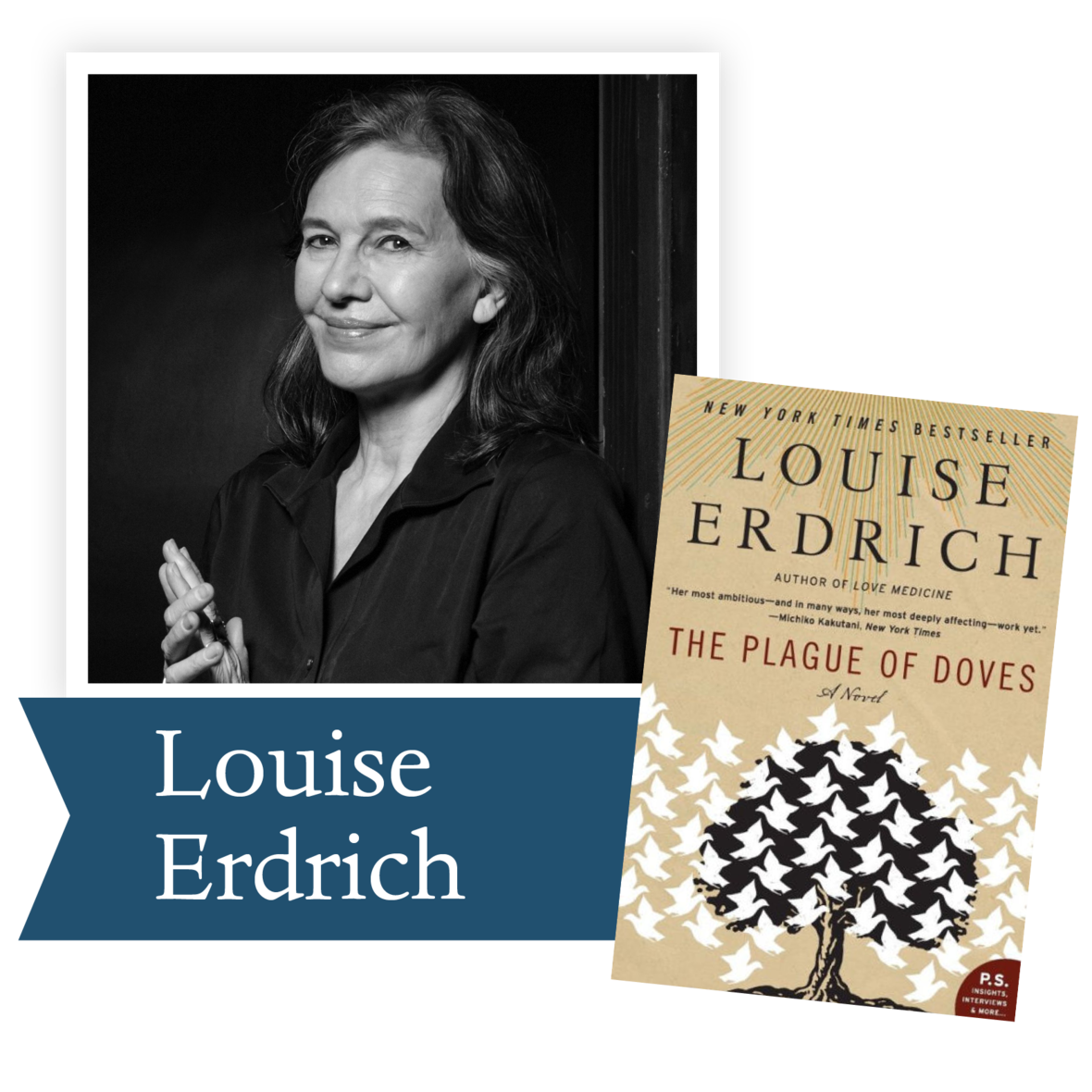The Plague of Doves by Louise Erdrich (Harper Collins): A violent act of racism haunts generations of Native American and white families living in rural North Dakota.

 table div table+table+table+table+table+table+table+table+table+table+table+table+table+table+table+table div table{width:100%;padding:0}table div table+table+table+table+table+table+table+table+table+table+table+table+table+table+table+table div table img{width:96.23%;padding:0;float:none}table div table+table+table+table+table+table+table+table+table+table+table+table+table+table+table+table div table td{width:100%;padding:0 1.88% 18px}/* styles */Beijing Coma by Ma Jian (Farrar, Straus and Giroux): Emerging from a coma caused by a bullet during the Tiananmen Square protests ten years earlier, a man recounts the horrors of the Mao era and senses the massive changes underway in China.

 table div table+table+table+table+table+table+table+table+table+table+table+table+table+table+table+table+table+table div table{width:100%;padding:0}table div table+table+table+table+table+table+table+table+table+table+table+table+table+table+table+table+table+table div table img{width:96.23%;padding:0;float:none}table div table+table+table+table+table+table+table+table+table+table+table+table+table+table+table+table+table+table div table td{width:100%;padding:0 1.88% 18px}/* styles */Telex from Cuba by Rachel Kushner (Scribner): The story of American executives and their families driven out of Cuba in 1958, Kushner’s powerful debut novel is a riveting exploration of colonialism, corporate America, and revolution.

 table div table+table+table+table+table+table+table+table+table+table+table+table+table+table+table+table+table+table+table+table div table{width:100%;padding:0}table div table+table+table+table+table+table+table+table+table+table+table+table+table+table+table+table+table+table+table+table div table img{width:96.23%;padding:0;float:none}table div table+table+table+table+table+table+table+table+table+table+table+table+table+table+table+table+table+table+table+table div table td{width:100%;padding:0 1.88% 18px}/* styles */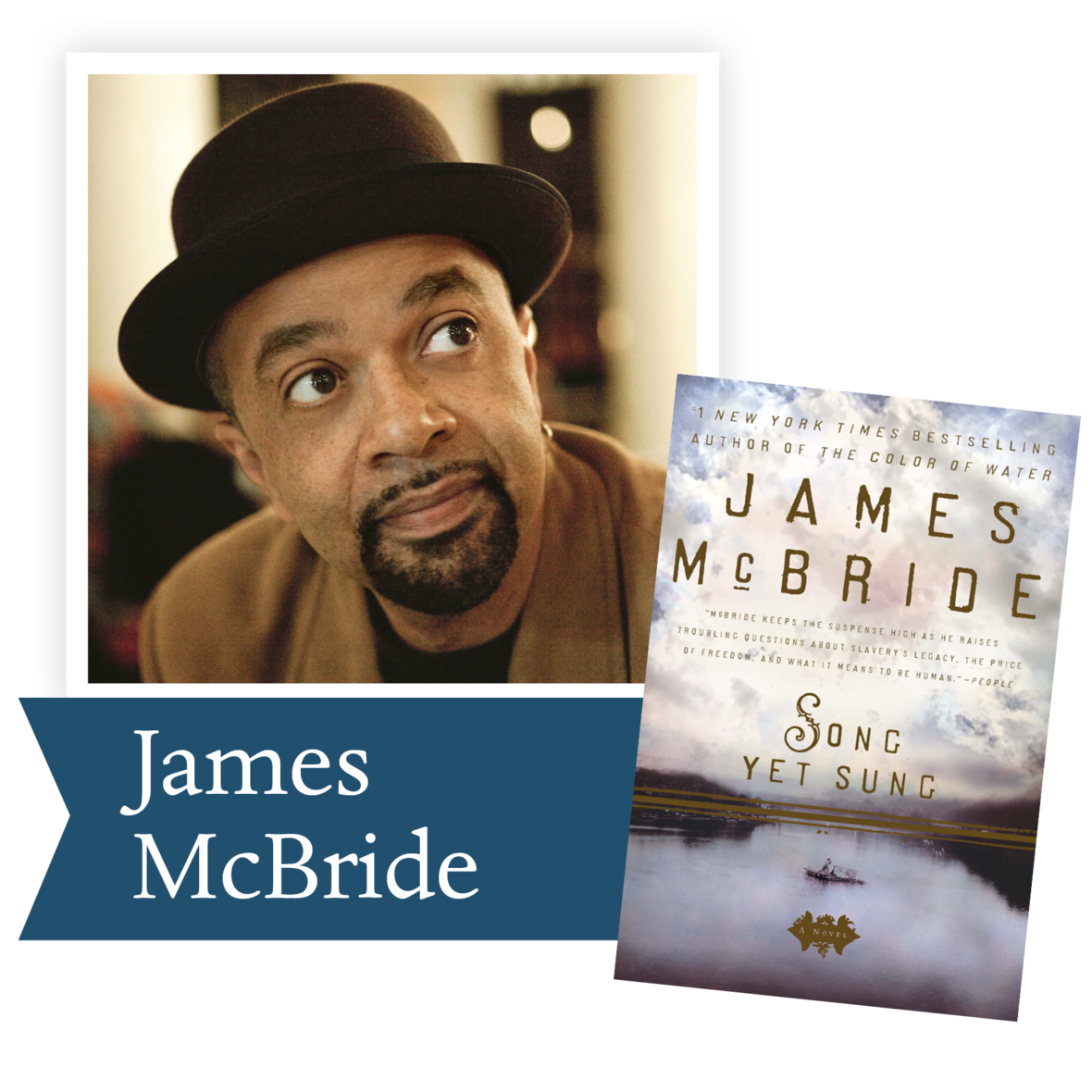Song Yet Sung by James McBride (Penguin Group): The haunting story of a runaway slave and a determined slave-catcher in pre-Civil War Maryland, Song Yet Sung explores both the moral choices faced by both blacks and whites and the meaning of freedom.

 table div table+table+table+table+table+table+table+table+table+table+table+table+table+table+table+table+table+table+table+table+table+table div table{width:100%;padding:0}table div table+table+table+table+table+table+table+table+table+table+table+table+table+table+table+table+table+table+table+table+table+table div table img{width:96.23%;padding:0;float:none}table div table+table+table+table+table+table+table+table+table+table+table+table+table+table+table+table+table+table+table+table+table+table div table td{width:100%;padding:0 1.88% 18px}/* styles */## 2009 Finalists - Nonfiction

 table div table+table+table+table+table+table+table+table+table+table+table+table+table+table+table+table+table+table+table+table+table+table+table+table div table{width:100%;padding:0}table div table+table+table+table+table+table+table+table+table+table+table+table+table+table+table+table+table+table+table+table+table+table+table+table div table img{width:96.23%;padding:0;float:none}table div table+table+table+table+table+table+table+table+table+table+table+table+table+table+table+table+table+table+table+table+table+table+table+table div table td{width:100%;padding:0 1.88% 18px}/* styles */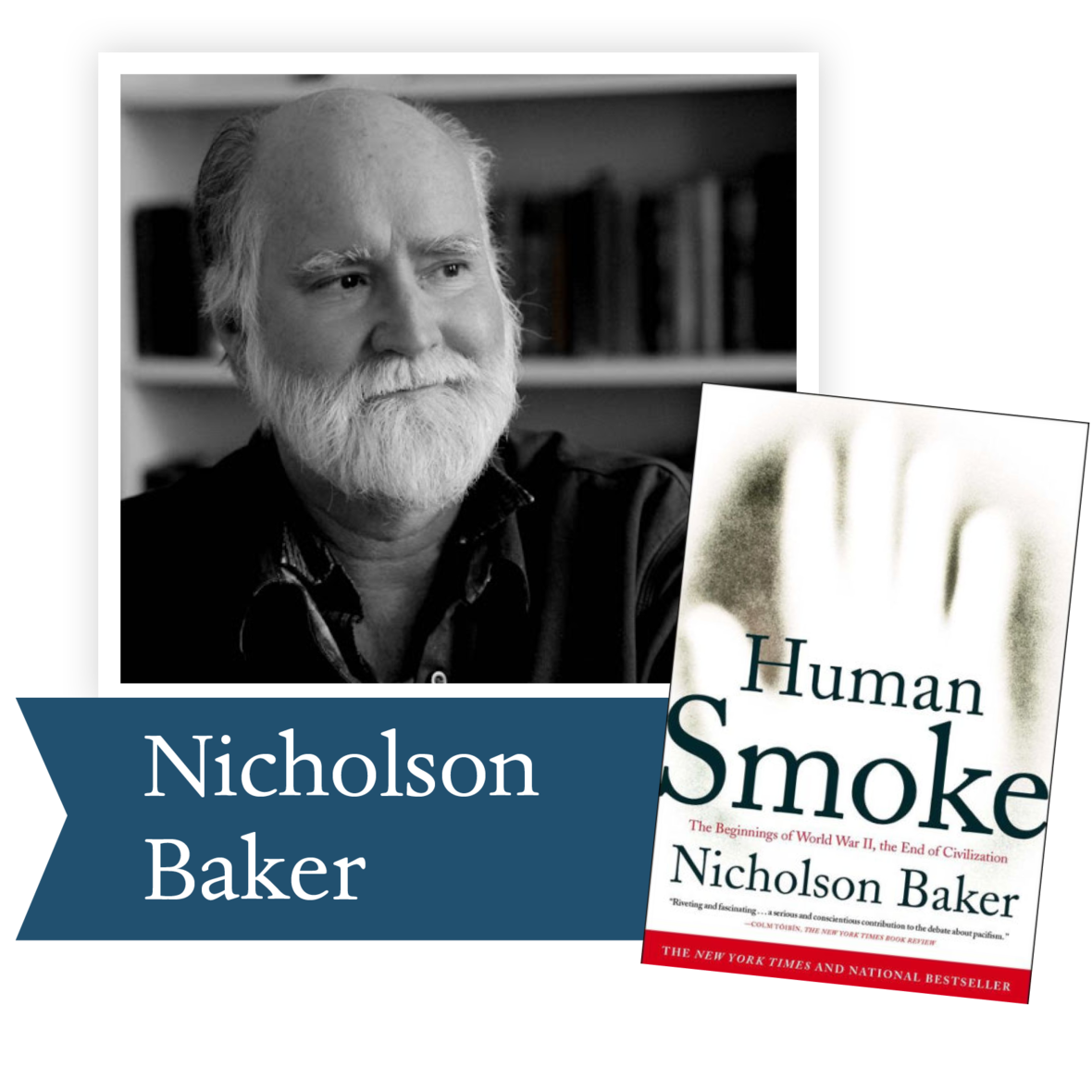Human Smoke: The Beginnings of World War II, the End of Civilization by Nicholson Baker (Simon & Schuster): In this wide-ranging, fresh perspective on the political and social landscape that gave rise to World War II, Baker makes a clear, compelling case in defense of pacifism.

 table div table+table+table+table+table+table+table+table+table+table+table+table+table+table+table+table+table+table+table+table+table+table+table+table+table+table div table{width:100%;padding:0}table div table+table+table+table+table+table+table+table+table+table+table+table+table+table+table+table+table+table+table+table+table+table+table+table+table+table div table img{width:96.23%;padding:0;float:none}table div table+table+table+table+table+table+table+table+table+table+table+table+table+table+table+table+table+table+table+table+table+table+table+table+table+table div table td{width:100%;padding:0 1.88% 18px}/* styles */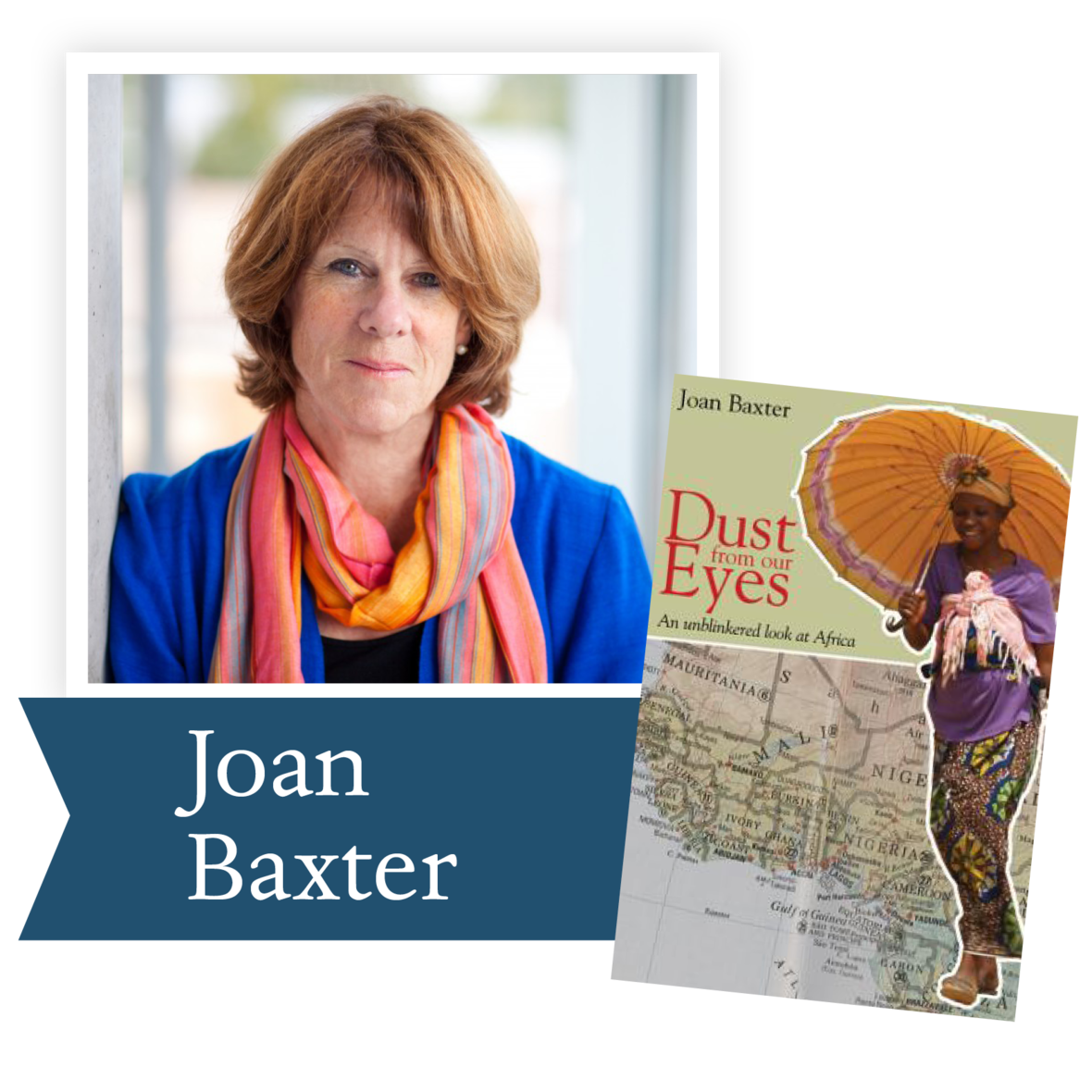Dust from our Eyes: An Unblinkered Look at Africa by Joan Baxter (Wolsak & Wynn): Baxter draws on more than two decades of living in and reporting from Africa to reveal that there is more to the continent than poverty and suffering, and far more to Western involvement than benevolent charity.

 table div table+table+table+table+table+table+table+table+table+table+table+table+table+table+table+table+table+table+table+table+table+table+table+table+table+table+table+table div table{width:100%;padding:0}table div table+table+table+table+table+table+table+table+table+table+table+table+table+table+table+table+table+table+table+table+table+table+table+table+table+table+table+table div table img{width:96.23%;padding:0;float:none}table div table+table+table+table+table+table+table+table+table+table+table+table+table+table+table+table+table+table+table+table+table+table+table+table+table+table+table+table div table td{width:100%;padding:0 1.88% 18px}/* styles */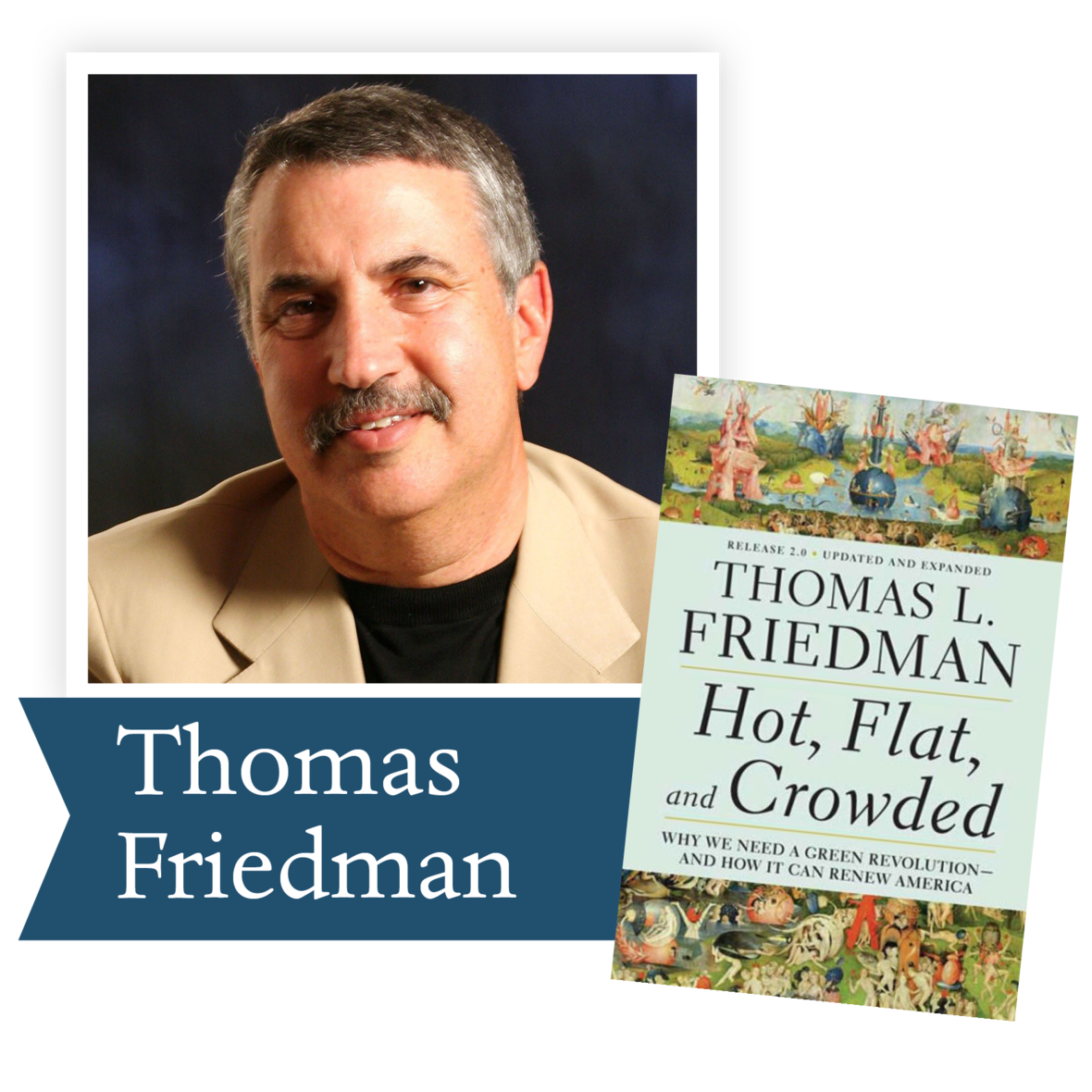Hot, Flat and Crowded by Thomas Friedman (Farrar, Straus & Giroux): Taking a provocative look at the crises of climate change and rising competition for energy, Friedman proposes a national strategy to make America healthier, richer, and more secure.

 table div table+table+table+table+table+table+table+table+table+table+table+table+table+table+table+table+table+table+table+table+table+table+table+table+table+table+table+table+table+table div table{width:100%;padding:0}table div table+table+table+table+table+table+table+table+table+table+table+table+table+table+table+table+table+table+table+table+table+table+table+table+table+table+table+table+table+table div table img{width:96.23%;padding:0;float:none}table div table+table+table+table+table+table+table+table+table+table+table+table+table+table+table+table+table+table+table+table+table+table+table+table+table+table+table+table+table+table div table td{width:100%;padding:0 1.88% 18px}/* styles */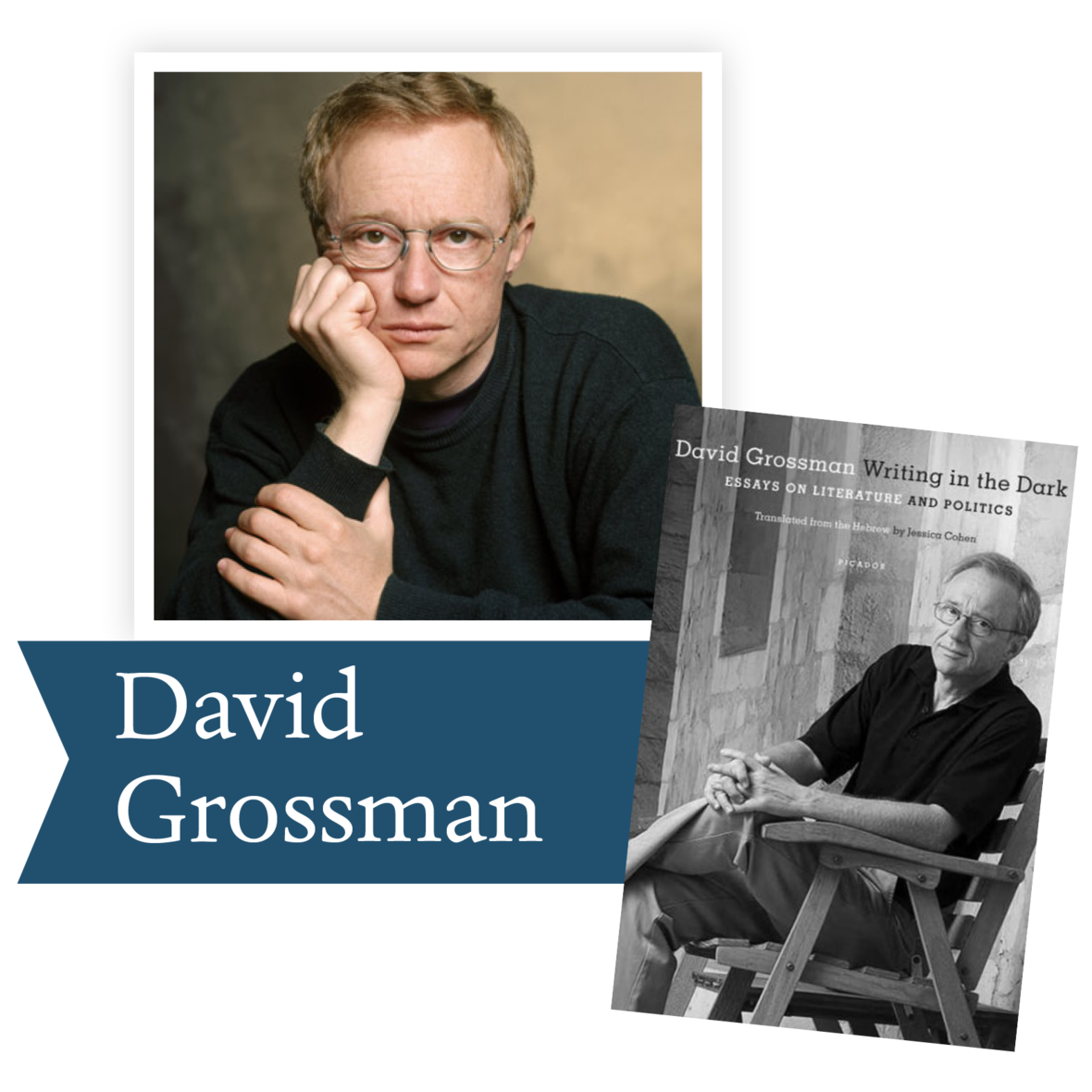Writing in the Dark by David Grossman (Farrar, Straus and Giroux): In six essays on politics and culture in Israel, including his speech on the 2006 Lebanon War, which took the life of his son, Grossman addresses the conscience of a country that has lost faith in its leaders and its ideals.

 table div table+table+table+table+table+table+table+table+table+table+table+table+table+table+table+table+table+table+table+table+table+table+table+table+table+table+table+table+table+table+table+table div table{width:100%;padding:0}table div table+table+table+table+table+table+table+table+table+table+table+table+table+table+table+table+table+table+table+table+table+table+table+table+table+table+table+table+table+table+table+table div table img{width:96.23%;padding:0;float:none}table div table+table+table+table+table+table+table+table+table+table+table+table+table+table+table+table+table+table+table+table+table+table+table+table+table+table+table+table+table+table+table+table div table td{width:100%;padding:0 1.88% 18px}/* styles */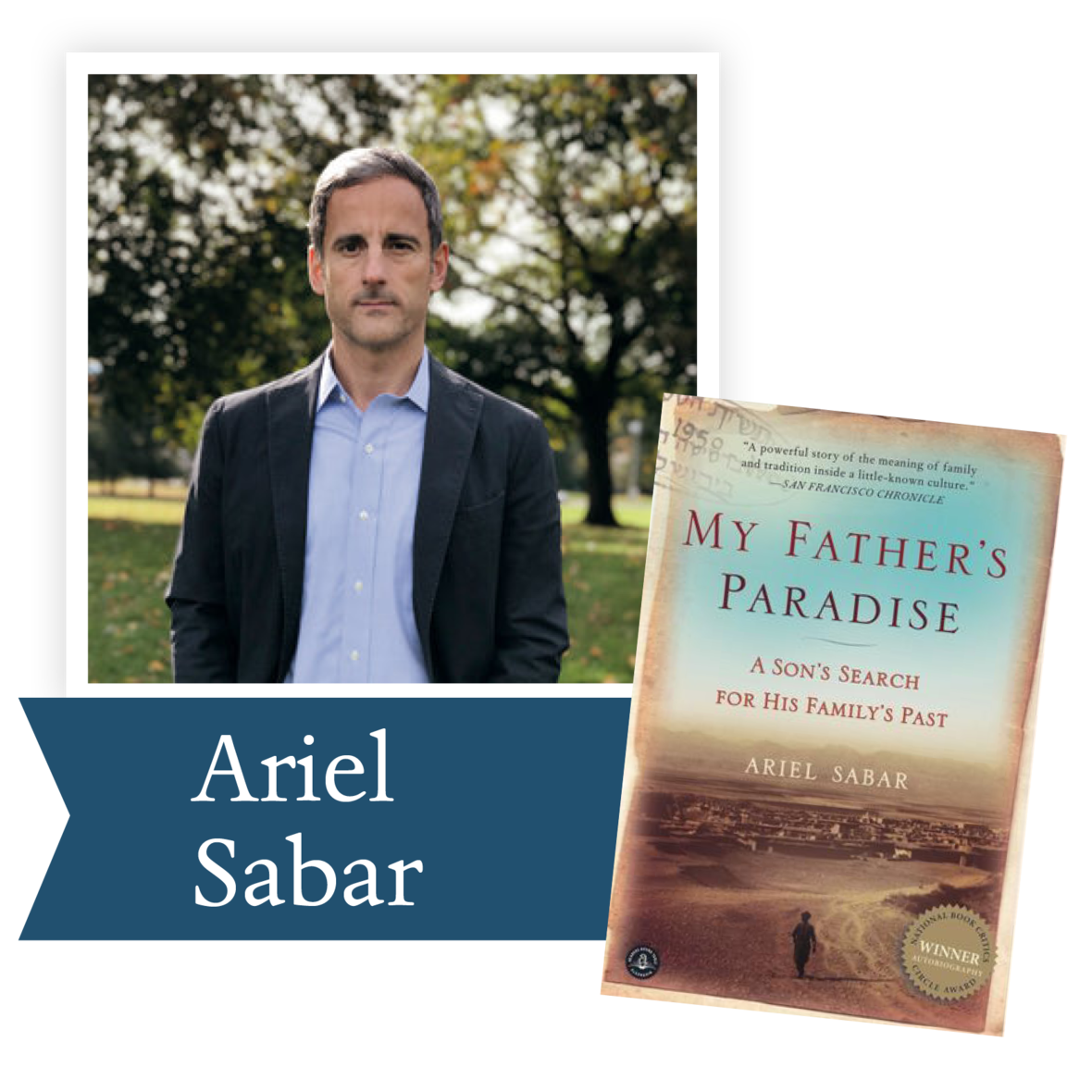My Father’s Paradise: A Son’s Search for his Father’s Past by Ariel Sabar (Algonquin): Traveling with his father to a remote corner of war-torn Iraq in a quest for roots and reconciliation, Sabar shares an intimate story of tolerance and hope in an Iraq very different from the one in the headlines today.

 table div table+table+table+table+table+table+table+table+table+table+table+table+table+table+table+table+table+table+table+table+table+table+table+table+table+table+table+table+table+table+table+table+table+table div table{width:100%;padding:0}table div table+table+table+table+table+table+table+table+table+table+table+table+table+table+table+table+table+table+table+table+table+table+table+table+table+table+table+table+table+table+table+table+table+table div table img{width:96.23%;padding:0;float:none}table div table+table+table+table+table+table+table+table+table+table+table+table+table+table+table+table+table+table+table+table+table+table+table+table+table+table+table+table+table+table+table+table+table+table div table td{width:100%;padding:0 1.88% 18px}/* styles */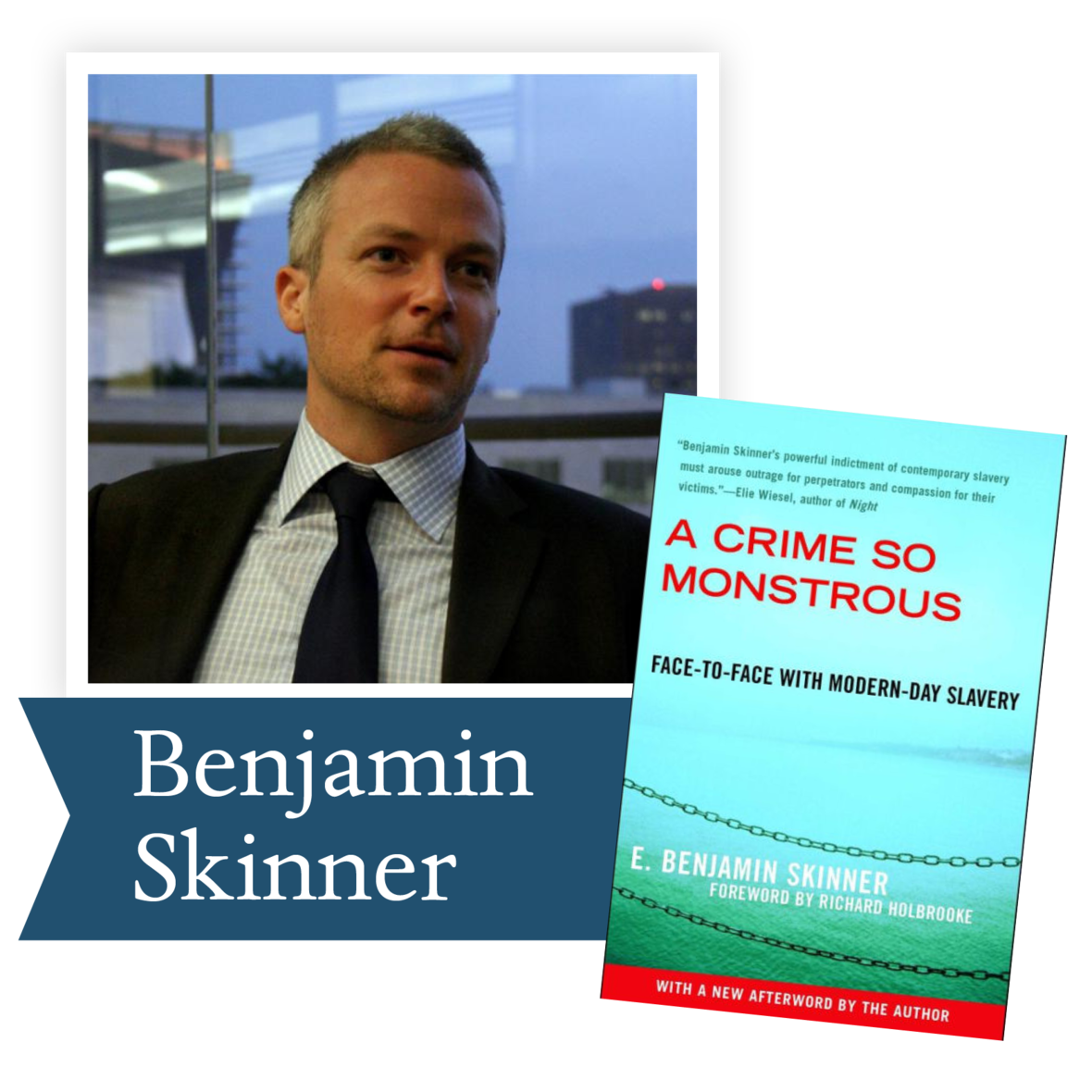A Crime So Monstrous: Face to Face with Modern Day Slavery by Benjamin Skinner (Free Press): Based on years of reporting in such places as Haiti, Sudan, India, Eastern Europe, The Netherlands, and, even suburban America, Skinner has produced a vivid testament and moving reportage on the horrors of contemporary slavery.

 table div table+table+table+table+table+table+table+table+table+table+table+table+table+table+table+table+table+table+table+table+table+table+table+table+table+table+table+table+table+table+table+table+table+table+table+table div table{width:100%;padding:0}table div table+table+table+table+table+table+table+table+table+table+table+table+table+table+table+table+table+table+table+table+table+table+table+table+table+table+table+table+table+table+table+table+table+table+table+table div table img{width:96.23%;padding:0;float:none}table div table+table+table+table+table+table+table+table+table+table+table+table+table+table+table+table+table+table+table+table+table+table+table+table+table+table+table+table+table+table+table+table+table+table+table+table div table td{width:100%;padding:0 1.88% 18px}/* styles */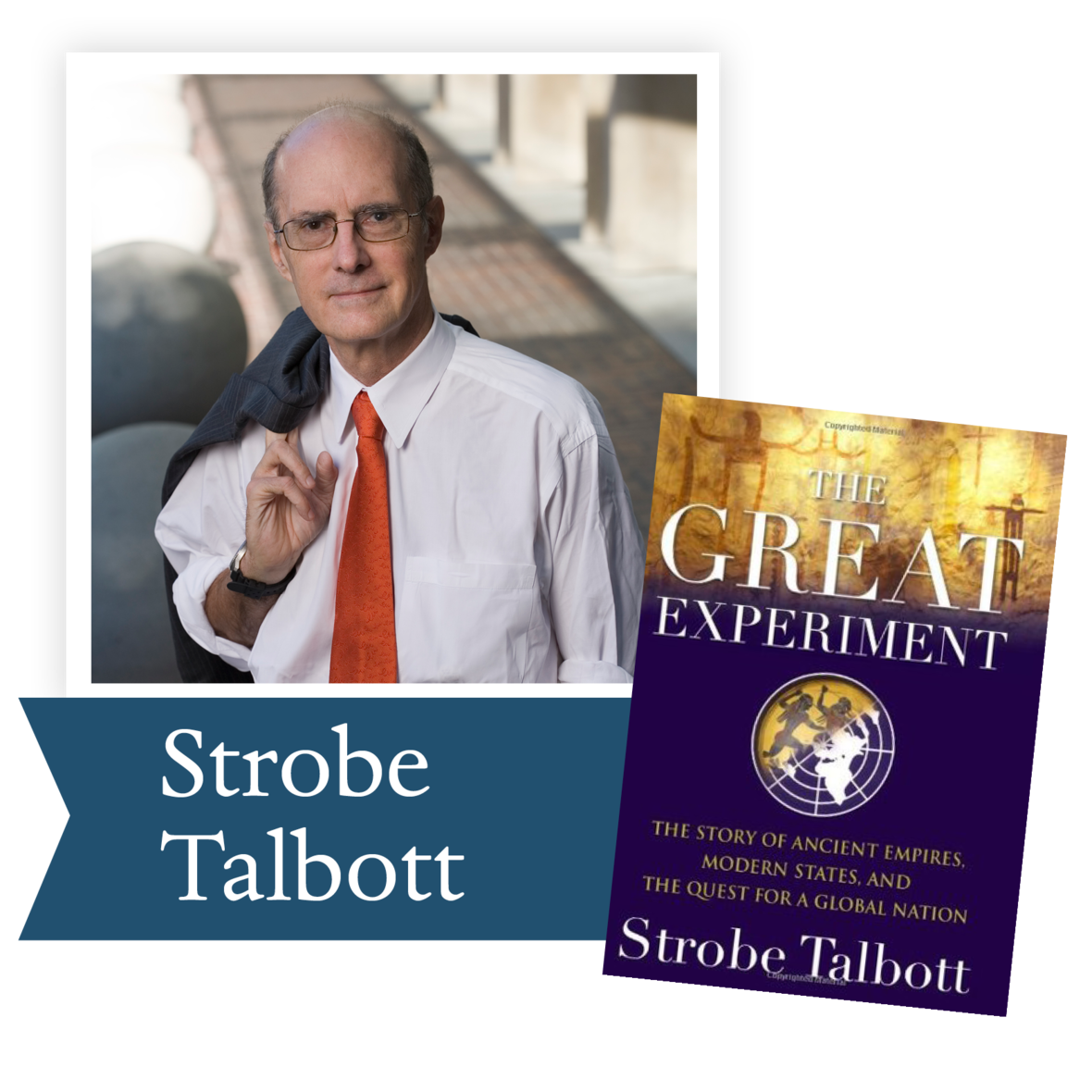The Great Experiment by Strobe Talbott (Simon & Schuster): Combining sweeping history with personal insight, Talbott explores the consolidation of tribes into nations and argues for America's unique role in modern history as "the master builder" of the international system.

 table div table+table+table+table+table+table+table+table+table+table+table+table+table+table+table+table+table+table+table+table+table+table+table+table+table+table+table+table+table+table+table+table+table+table+table+table+table+table div table{width:100%;padding:0}table div table+table+table+table+table+table+table+table+table+table+table+table+table+table+table+table+table+table+table+table+table+table+table+table+table+table+table+table+table+table+table+table+table+table+table+table+table+table div table img{width:96.23%;padding:0;float:none}table div table+table+table+table+table+table+table+table+table+table+table+table+table+table+table+table+table+table+table+table+table+table+table+table+table+table+table+table+table+table+table+table+table+table+table+table+table+table div table td{width:100%;padding:0 1.88% 18px}/* styles */## 2009 Lifetime Achievement Award Winner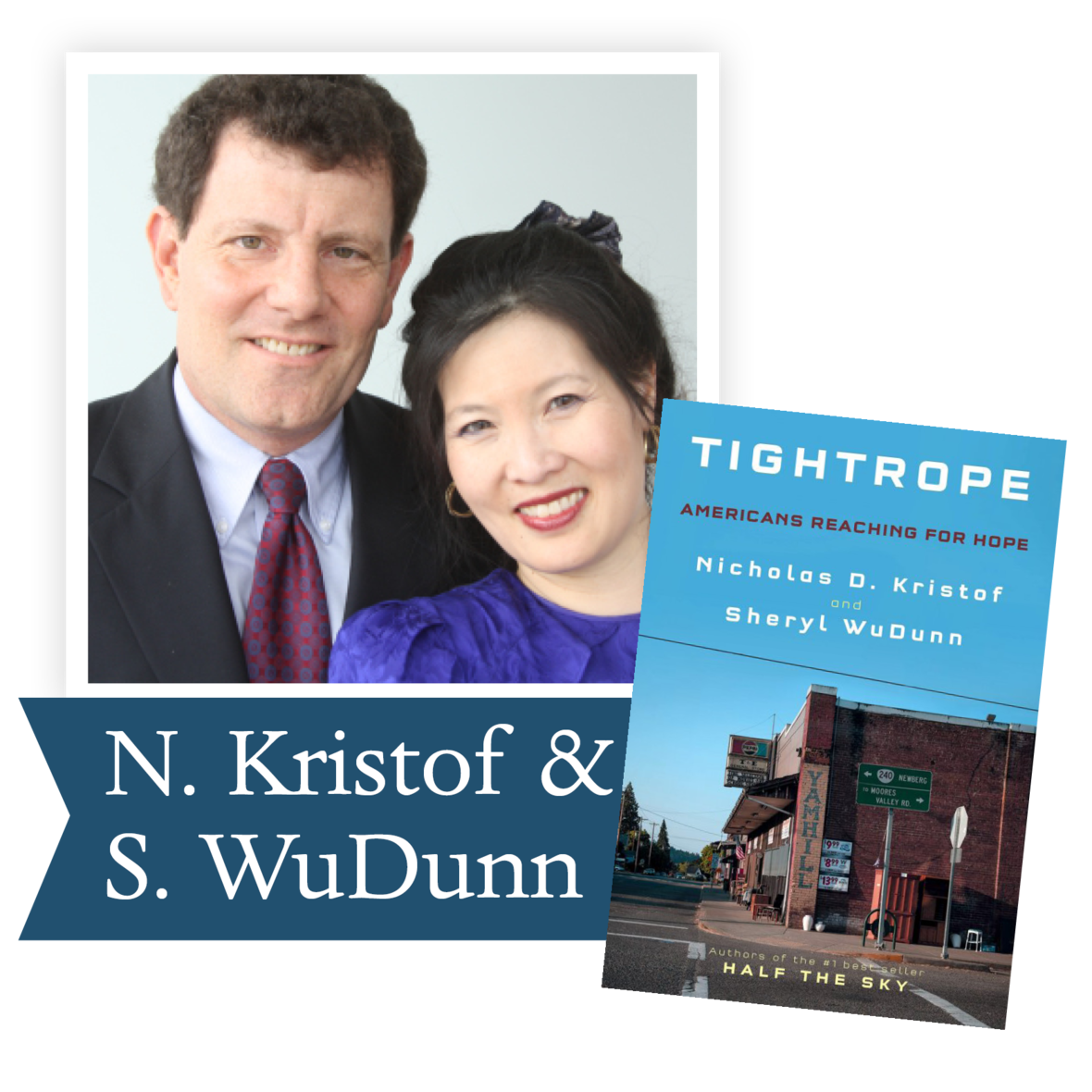Today we are using a PBS site to highlight Nicholas Kristoff and Sheryl WuDunn and their extraordinary careers.

However, the PBS program was filmed to introduce their award-winning Holding Up Half the Sky. We would like to also share with you the the publication of their newest book, Tightrope: Americans Reaching for Hope. Our former DLPP Winner Dave Eggers wrote this review:

“This is an unflinching book that illustrates the central, confounding American paradox—in a country that purports to root for the underdog, too often we exalt the rich and we punish the poor. With thorough reporting and extraordinary compassion, Kristof and WuDunn tell the stories of those who fall behind in the world’s wealthiest country, and find not an efficient first-world safety net created by their government, but a patchwork of community initiatives, perpetually underfunded and run by tired saints. And yet amid all the tragedy and neglect, Kristof and WuDunn conjure a picture of how it could all get better, how it could all work. That’s the miracle of Tightrope, and why this is such an indispensable book.” —Dave Eggers, 2010 DLPP Nonfiction Winner for Zeitoun

 table div table+table+table+table+table+table+table+table+table+table+table+table+table+table+table+table+table+table+table+table+table+table+table+table+table+table+table+table+table+table+table+table+table+table+table+table+table+table+table+table+table div table{width:100%;padding:0}table div table+table+table+table+table+table+table+table+table+table+table+table+table+table+table+table+table+table+table+table+table+table+table+table+table+table+table+table+table+table+table+table+table+table+table+table+table+table+table+table+table div table img{width:96.23%;padding:0;float:none}table div table+table+table+table+table+table+table+table+table+table+table+table+table+table+table+table+table+table+table+table+table+table+table+table+table+table+table+table+table+table+table+table+table+table+table+table+table+table+table+table+table div table td{width:100%;padding:0 1.88% 18px}/* styles */table div table+table+table+table+table+table+table+table+table+table+table+table+table+table+table+table+table+table+table+table+table+table+table+table+table+table+table+table+table+table+table+table+table+table+table+table+table+table+table+table+table+table+table div table{width:100%;padding:0}table div table+table+table+table+table+table+table+table+table+table+table+table+table+table+table+table+table+table+table+table+table+table+table+table+table+table+table+table+table+table+table+table+table+table+table+table+table+table+table+table+table+table+table div table img{width:96.23%;padding:0;float:none}table div table+table+table+table+table+table+table+table+table+table+table+table+table+table+table+table+table+table+table+table+table+table+table+table+table+table+table+table+table+table+table+table+table+table+table+table+table+table+table+table+table+table+table div table td{width:100%;padding:0 1.88% 18px}/* styles */Jesmyn Ward, a three-time finalist for the Dayton Literary Peace Prize, won the National Book Award for Sing, Unburied, Sing. Her work can educate us about the racial issues facing America today. Please join us in July to discuss this important book.

“The heart of Jesmyn Ward’s Sing, Unburied, Sing is story—the yearning for a narrative to help us understand ourselves, the pain of the gaps we’ll never fill, the truths that are failed by words and must be translated through ritual and song...Ward’s writing throbs with life, grief, and love, and this book is the kind that makes you ache to return to it.” —Buzzfeed

Ron Rollins, recently retired Dayton Daily News Ideas and Voices Editor, will moderate the discussion.

 table div table+table+table+table+table+table+table+table+table+table+table+table+table+table+table+table+table+table+table+table+table+table+table+table+table+table+table+table+table+table+table+table+table+table+table+table+table+table+table+table+table+table+table+table+table div table{width:100%;padding:0}table div table+table+table+table+table+table+table+table+table+table+table+table+table+table+table+table+table+table+table+table+table+table+table+table+table+table+table+table+table+table+table+table+table+table+table+table+table+table+table+table+table+table+table+table+table div table img{width:96.23%;padding:0;float:none}table div table+table+table+table+table+table+table+table+table+table+table+table+table+table+table+table+table+table+table+table+table+table+table+table+table+table+table+table+table+table+table+table+table+table+table+table+table+table+table+table+table+table+table+table+table div table td{width:100%;padding:0 1.88% 18px}/* styles */